# Climb in percentage

The height difference between points A and B is 475 m. Calculate the percentage of route climbing if the horizontal distance places A, B is 7.4 km.

Correct result:

p =  6.42 %

#### Solution:

$y=475 \ \text{m} \ \\ x=7.4 \ km \rightarrow m=7.4 \cdot \ 1000 \ m=7400 \ m \ \\ \ \\ p=100 \cdot \ \dfrac{ y }{ x }=100 \cdot \ \dfrac{ 475 }{ 7400 }=\dfrac{ 475 }{ 74 }=6.42 \%$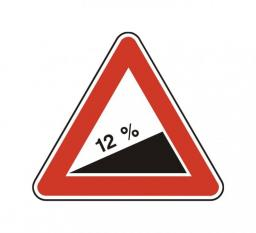Our examples were largely sent or created by pupils and students themselves. Therefore, we would be pleased if you could send us any errors you found, spelling mistakes, or rephasing the example. Thank you!

Please write to us with your comment on the math problem or ask something. Thank you for helping each other - students, teachers, parents, and problem authors.Tips to related online calculators
Check out our ratio calculator.
Do you want to convert length units?

#### You need to know the following knowledge to solve this word math problem:

We encourage you to watch this tutorial video on this math problem:

## Next similar math problems:

• Traffic signThere is a traffic sign for climbing on the road with an angle of 7%. Calculate at what angle the road rises (falls).
• In the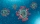In the workshop, it was necessary to quickly complete the production of veils for the hospital. The workshop staff promised to work an additional 240 hours to produce this order in March. The workshop has 8 employees who work 160 hours a month. What perce
• Water percent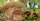A 15 g sample of the substance contains 70% water. After drying, the weight was reduced to 9 g. What percentage of water is now in the sample?
• What isWhat is the annual percentage increase in the city when the population has tripled in 20 years?
• Price reductionThe product is sold for 360 CZK and the sales profit is 30%. By what percentage the sales profit will be reduced if I reduce the price of the product by 10%?
• Metal sheets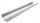How much metal sheet is needed to produce 8 gutters 4 m long and 12 cm in diameter? During production joints calculate at 3% of total consumption.
• Hazard game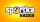In the Sportka hazard game, 6 numbers out of 49 are drawn. What is the probability that we will win: a) second prize (we guess 5 numbers correctly) b) the third prize (we guess 4 numbers correctly)?
• Hiking tripRosie went on a hiking trip. The first day she walked 18kilometers. Each day since she walked 90 percent of what she walked the day before. What is the total distance Rosie has traveled by the end of the 10th day? Round your final answer to the nearest ki
• Beer tapping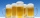When checking compliance with the beer tapping, it was found that 60% of the offered beers were underfilled. The others were fine. Thus, instead of 0.5 l, the volume was 4.4 dcl on average. What was the volume of one average underfilled beer?
• Bike cost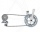The father gave his son € 100 to buy a bicycle, which was 40% of the total amount of the bicycle. How much did the bike cost?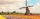How large must the group of people be so that the probability that two people have a birthday on the same day of the year is greater than 90%?How long will it take to save € 9,000 by depositing € 200 at the beginning of each year at 2% interest?The number 72 increase by 25%. By how much % will you have to reduce the number you created to get the number 72 again?It is reported that 72% of working women use computers at work. Choose 3 women at random, find the probability that all 3 women use a computer in their jobs.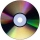The manufacturer found that 3% of the plates produced had a malfunction. Of the compliant, 75% are first and 25% second. What is the probability of producing first and second class plates?How probable is a randomly selected three-digit number divisible by five or seven?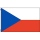The children had the club flag sewn on. The red color covered 2/20 of the total area of the flag, the blue area a fifth, the yellow 19% of the total area. What% is the green area occupied?